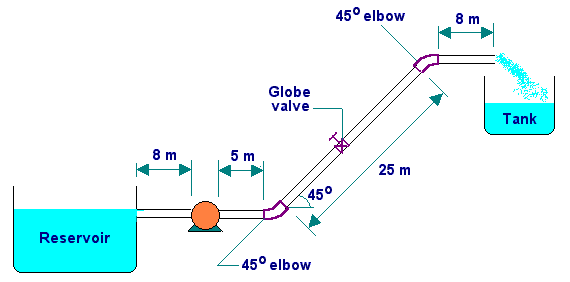4HE - Fluid Mechanics     Test - 2 (March-2001)      Maximum Marks : 60     Duration : 90 minutes

Part A (12 x 2 = 24 Marks)
1. With a neat sketch, compare the velocity profiles for laminar and turbulent flow of a fluid in a pipeline.
2. Indicate the methods to reduce (i) skin frictional losses
(ii) form frictional losses.
3. Calculate the hydraulic radius for flow of fluid through a rectangular duct of size 0.5 m x 0.3 m.
4. With a neat diagram, illustrate the relation between friction factor and Reynolds number, for laminar and turbulent flows in circular ducts, indicating the effect of roughness.
5. The relation between frictional pressure drop (Dp) and volumetric flow rate (Q) for flow through pipelines is given by Dp = cQn ; where c is a constant. What is the value of 'n' for (i) laminar flow
(ii) turbulent flow through very smooth pipe
(iii) turbulent flow through very rough pipe
6. Draw the shape of nozzles, that is needed for accelerating the flow velocity, for the following flow conditions, indicating the flow direction: (i) subsonic flow to soinc flow
(ii) sonic to supersonic flow
(iii) subsonic to supersonic flow
7. Draw a neat sketch showing the development of boundary layer for laminar flow in pipe, also indicate the shape of the velocity profiles at developing and developed sections.
8. How does the pressure drop vary with the flow rate in a rota-meter, and in orifice meter?
9. The pressure drop between upstream and throat of venturi meter is 100 cm of the flowing fluid. What is the pressure drop if the volumetric flow rate is doubled?
10. Compare the manometer readings of orifice meter and venturi meter for a flowrate of Q through a pipe of diameter D, given that diameter of venturi throat = diameter of orifice opening.
11. Give two industrial applications of: (i) packed beds
(ii) fluidized beds
12. Differentiate between aggregative and particulate fluidization.
13. Part B (3 x 12 = 36 Marks)
14. (a) By applying momentum balance to the steady flow of a fluid in a stream-tube, derive Eulers equation of motion. (8)
(b) Obtain Bernoulli equation from Euler equation by making necessary assumptions. (4)
Or
15. For laminar flow of a Newtonian fluid in circular pipe, obtain the following relations from first principles: (i) Frictional pressure drop and wall shear stress
(ii) Velocity distribution in the radial direction
(iii) Average velocity and maximum velocity
(iv) Pressure drop and average velocity

1. Water is pumped from a ground level reservoir to an overhead tank through a 7.5 cm ID pipe as shown in the sketch:(a) What pressure is needed at the outlet of the pump to supply water to the tank at the rate of 100 ltr/min?
(b) What is the power required for the pump, if the pump is only 60% efficient?
Data:
m = 1 cP; r = 1 g/ml.
Equivalent length of fittings (Le/D):
Globe valve (open) : 300
45o elbow : 15
Fanning friction factor for turbulent flow is given by: f = 0.079 (NRe)-0.25

2. Or
3. (a) A venturi meter has throat to upstream pipe cross-section ratio of 0.5. The fluid flowing is water. The pressure at the entry of converging cone section is 137.9 kN/m2(a). What is the velocity at the throat which corresponds to a pressure of 0 kN/m2(a) at the throat? If the water is at 200oF, what is the highest velocity possible at the throat at which water will boil?
Data: For water at 200oF,
Density = 980 kg/m3. Vapor pressure = 75.8 kN/m2(a).                (8)

(b) A rotameter with a stainless steel float has a maximum capacity of 1.2 ltr/sec of water. What will be the maximum capacity for kerosene in ltr/sec for the same rotameter and float? Assume that the Cd for the rotameter is not changing much with flowrate.
Data:
Specific gravity of stainless steel = 7.92
Specifc gravity of kerosene = 0.82                (4)

4. (a) Derive relations for Reynolds number and friction factor and establish the Ergun equation for single-phase flow of fluid through packed bed. (8)
(b) Bring out the equation predicting minimum fluidization velocity. (4)

5. Or
6. A mixture of vapors pass through a packed bed of glass spheres having density 2.4 g/cc each of diameter 0.5 cm. The pressure drop due to the flow is 4000 N/m2. The height of packed bed is 1.85 m. The density and viscosity of the vapor mixture are 3.8 x 10-3 g/cc and 0.015 cP respectively.
Data:
Cross sectional area of the packed column tube = 0.09 m2
Bed porosity = 0.4

Find the mass flow rate of the vapor mixture.